# Source Separation with Sparsity¶

Important: Please read the installation page for details about how to install the toolboxes. $\newcommand{\dotp}{\langle #1, #2 \rangle}$ $\newcommand{\enscond}{\lbrace #1, #2 \rbrace}$ $\newcommand{\pd}{ \frac{ \partial #1}{\partial #2} }$ $\newcommand{\umin}{\underset{#1}{\min}\;}$ $\newcommand{\umax}{\underset{#1}{\max}\;}$ $\newcommand{\umin}{\underset{#1}{\min}\;}$ $\newcommand{\uargmin}{\underset{#1}{argmin}\;}$ $\newcommand{\norm}{\|#1\|}$ $\newcommand{\abs}{\left|#1\right|}$ $\newcommand{\choice}{ \left\{ \begin{array}{l} #1 \end{array} \right. }$ $\newcommand{\pa}{\left(#1\right)}$ $\newcommand{\diag}{{diag}\left( #1 \right)}$ $\newcommand{\qandq}{\quad\text{and}\quad}$ $\newcommand{\qwhereq}{\quad\text{where}\quad}$ $\newcommand{\qifq}{ \quad \text{if} \quad }$ $\newcommand{\qarrq}{ \quad \Longrightarrow \quad }$ $\newcommand{\ZZ}{\mathbb{Z}}$ $\newcommand{\CC}{\mathbb{C}}$ $\newcommand{\RR}{\mathbb{R}}$ $\newcommand{\EE}{\mathbb{E}}$ $\newcommand{\Zz}{\mathcal{Z}}$ $\newcommand{\Ww}{\mathcal{W}}$ $\newcommand{\Vv}{\mathcal{V}}$ $\newcommand{\Nn}{\mathcal{N}}$ $\newcommand{\NN}{\mathcal{N}}$ $\newcommand{\Hh}{\mathcal{H}}$ $\newcommand{\Bb}{\mathcal{B}}$ $\newcommand{\Ee}{\mathcal{E}}$ $\newcommand{\Cc}{\mathcal{C}}$ $\newcommand{\Gg}{\mathcal{G}}$ $\newcommand{\Ss}{\mathcal{S}}$ $\newcommand{\Pp}{\mathcal{P}}$ $\newcommand{\Ff}{\mathcal{F}}$ $\newcommand{\Xx}{\mathcal{X}}$ $\newcommand{\Mm}{\mathcal{M}}$ $\newcommand{\Ii}{\mathcal{I}}$ $\newcommand{\Dd}{\mathcal{D}}$ $\newcommand{\Ll}{\mathcal{L}}$ $\newcommand{\Tt}{\mathcal{T}}$ $\newcommand{\si}{\sigma}$ $\newcommand{\al}{\alpha}$ $\newcommand{\la}{\lambda}$ $\newcommand{\ga}{\gamma}$ $\newcommand{\Ga}{\Gamma}$ $\newcommand{\La}{\Lambda}$ $\newcommand{\si}{\sigma}$ $\newcommand{\Si}{\Sigma}$ $\newcommand{\be}{\beta}$ $\newcommand{\de}{\delta}$ $\newcommand{\De}{\Delta}$ $\newcommand{\phi}{\varphi}$ $\newcommand{\th}{\theta}$ $\newcommand{\om}{\omega}$ $\newcommand{\Om}{\Omega}$

This numerical tour explore local Fourier analysis of sounds, and its application to source separation from stereo measurements.

In :
from __future__ import division

import numpy as np
import scipy as scp
import pylab as pyl
import matplotlib.pyplot as plt

from nt_toolbox.general import *
from nt_toolbox.signal import *

import warnings
warnings.filterwarnings('ignore')

%matplotlib inline


## Sound Mixing¶

We load 3 sounds and simulate a stero recording by performing a linear blending of the sounds.

In :
from nt_toolbox.load_sound import *

n = 1024*16
s = 3 #number of sounds
p = 2 #number of micros

x = np.zeros([n,3])


Normalize the energy of the signals.

In :
x = x/np.tile(np.std(x,0),(n,1))


We mix the sound using a $2\mathrm{x}3$ transformation matrix. Here the direction are well-spaced, but you can try with more complicated mixing matrices.

Compute the mixing matrix

In :
theta = np.linspace(0, np.pi, s + 1)[:-1]
theta = .2
M = np.vstack((np.cos(theta), np.sin(theta)))


Compute the mixed sources.

In :
y = np.dot(x,np.transpose(M))


Display of the sounds and their mix.

In :
plt.figure(figsize = (10,10))

for i in range(s):
plt.subplot(s, 1, i+1)
plt.plot(x[:, i])
plt.xlim(0,n)
plt.title("Source #%i" %(i+1))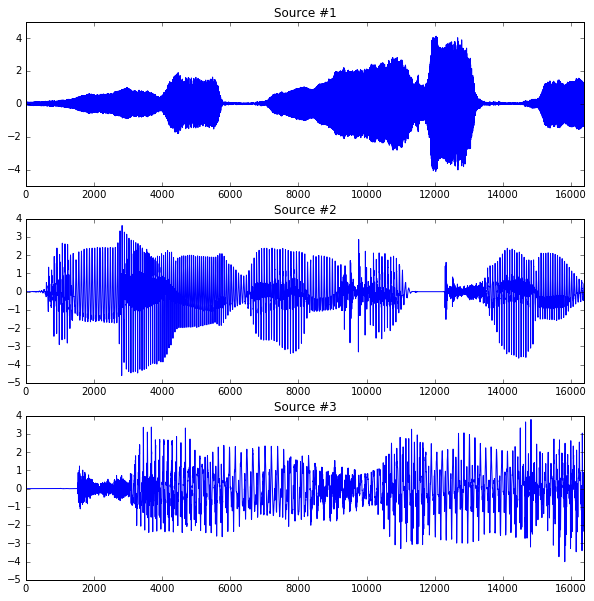Display of the micro output.

In :
plt.figure(figsize = (10,7))

for i in range(p):
plt.subplot(p, 1, i+1)
plt.plot(y[:, i])
plt.xlim(0,n)
plt.title("Micro #%i" %(i+1))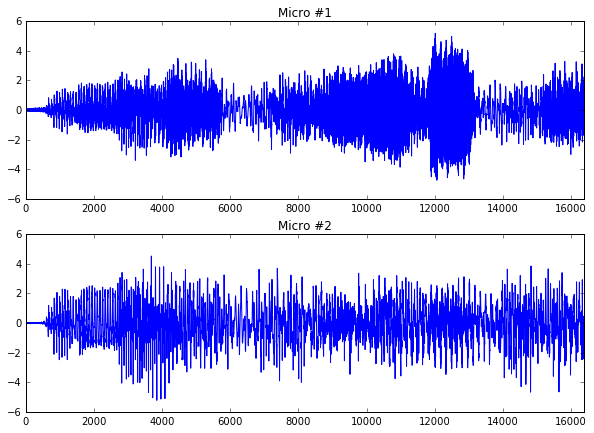## Local Fourier analysis of sound.¶

In order to perform the separation, one performs a local Fourier analysis of the sound. The hope is that the sources will be well-separated over the Fourier domain because the sources are sparse after a STFT.

First set up parameters for the STFT.

In :
w = 128   #size of the window
q = w//4  #overlap of the window


Compute the STFT of the sources.

In :
from nt_toolbox.perform_stft import *
from nt_toolbox.plot_spectrogram import *

X = np.zeros([w,4*w+1,s])
Y = np.zeros([w,4*w+1,p])

for i in range(s):
X[:,:,i] = perform_stft(x[:,i],w,q,n)
plt.figure(figsize = (15,10))
plot_spectrogram(X[:,:,i],"Source #%i" %(i+1))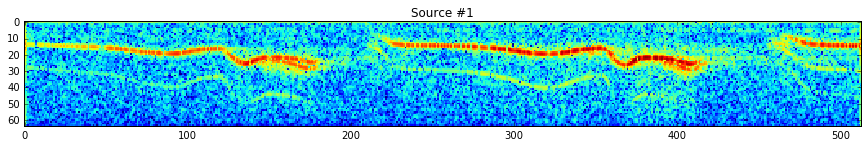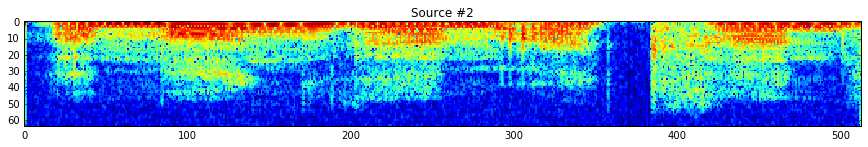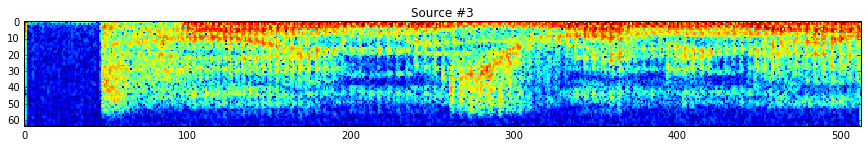Exercise 1

Compute the STFT of the micros, and store them into a matrix |Y|.

In :
run -i nt_solutions/audio_2_separation/exo1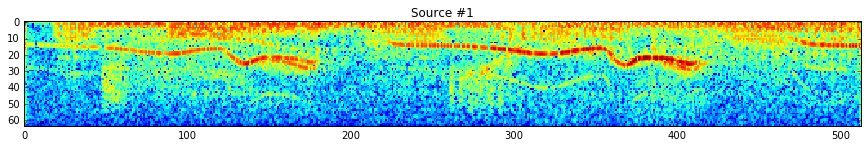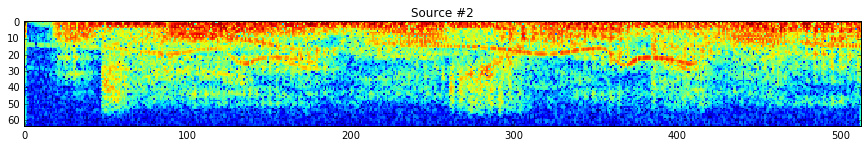In :
## Insert your code here.


## Estimation of Mixing Direction by Clustering¶

Since the sources are quite sparse over the Fourier plane, the directions are well estimated by looking as the direction emerging from a point clouds of the transformed coefficients.

First we compute the position of the point cloud.

In :
mf = np.shape(Y)
mt = np.shape(Y)
P = np.reshape(Y, (mt*mf,p))
P = np.vstack((np.real(P), np.imag(P)))


Then we keep only the 5% points with largest energy.

Display some points in the original (spacial) domain.

Number of displayed points.

In :
npts = 6000


Display the original points.

In :
from numpy import random

sel = random.permutation(n)

sel = sel[:npts]

plt.figure(figsize = (7,5))
plt.plot(y[sel,0], y[sel,1], ".", ms = 3)
plt.xlim(-5,5)
plt.ylim(-5,5)
plt.title('Time domain')
plt.show()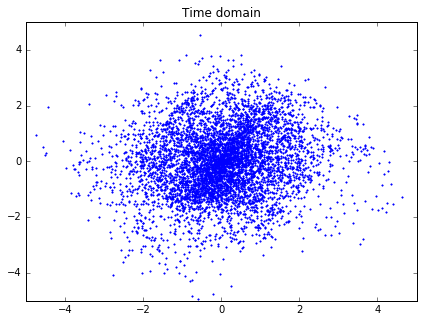Exercise 2

Display some points of $P$ in the transformed (time/frequency) domain.

In :
run -i nt_solutions/audio_2_separation/exo2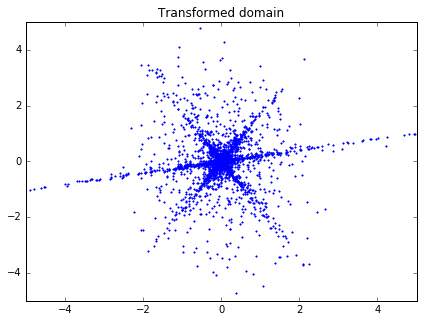In :
## Insert your code here.


We compute the angle associated to each point over the transformed domain. The histogram shows the main direction of mixing.

In :
import math

nrow = np.shape(P)
Theta = np.zeros(nrow)
for i in range(nrow):
Theta[i] = math.atan2(P[i,1],P[i,0])%np.pi


Display histogram.

In :
nbins = 100
t = np.linspace(np.pi/200,np.pi,nbins)
hist = np.histogram(Theta,t)
h = hist/np.sum(hist)
t = t[:-1]

plt.figure(figsize = (7,5))
plt.bar(t, h, width = np.pi/nbins, color = "darkblue", edgecolor = "darkblue")
plt.xlim(0,np.pi)
plt.ylim(0,np.max(h))
plt.show()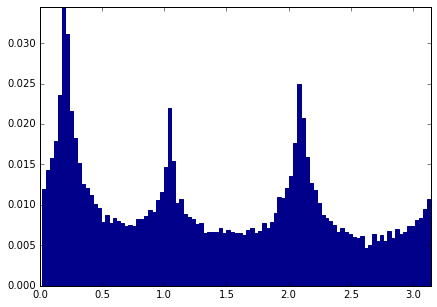Exercise 3

The histogram computed from the whole set of points are not peacked enough. To stabilize the detection of mixing direction, compute an histogram from a reduced set of point that have the largest amplitude. Compute the energy of each point. Extract only a small sub-set.

In :
run -i nt_solutions/audio_2_separation/exo3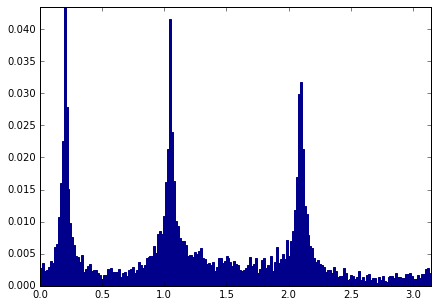In :
## Insert your code here.


Exercise 4

Detect the direction $M_1$ approximating the true direction $M$ by looking at the local maxima of the histogram. First detect the set of local maxima, and then keep only the three largest. Sort in descending order.

In :
run -i nt_solutions/audio_2_separation/exo4

--- M ---
[[ 0.98006658  0.5        -0.5       ]
[ 0.19866933  0.8660254   0.8660254 ]]
--- M1 ---
[[ 0.98190316  0.50797406 -0.49567393]
[ 0.18938372  0.86137237  0.8685087 ]]

In :
## Insert your code here.


## Separation of the Sources using Clustering¶

Once the mixing direction are known, one can project the sources on the direction.

We compute the projection of the coefficients Y on each estimated direction.

In :
A = np.reshape(Y, (mt*mf,p))


Compute the projection of the coefficients on the directions.

In :
C = abs(np.dot(np.transpose(M1),np.transpose(A)))


At each point $x$, the index $I(x)$ is the direction which creates the largest projection.

$I$ is the index of the closest source.

In :
tmp = np.max(C,0)
I = np.argmax(C,0)
I = np.reshape(I, (mf,mt)) + 1


An additional denoising is achieved by removing small coefficients.

In :
T = .05
D = np.sqrt(np.sum(abs(Y)**2, 2))
I = I*(D > T)


We can display the segmentation of the time frequency plane.

In :
plt.figure(figsize = (15,10))
imageplot(I[:mf//2,:])
plt.imshow(I[:mf//2,:], cmap = plt.get_cmap("jet"), interpolation = "nearest")
plt.show()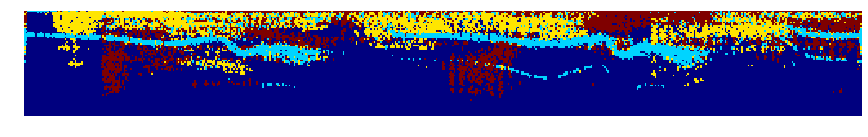The recovered coefficients are obtained by projection.

In :
Proj = np.dot(np.transpose(M1),np.transpose(A))
Xr = np.zeros([w,4*w+1,s])
for i in range(s):
Xr[:,:,i] = np.reshape(Proj[i,:], (mf,mt))*(I == i)


The estimated signals are obtained by inverting the STFT.

In :
xr = np.zeros([n,s])
for i in range(s):
xr[:,i] = perform_stft(Xr[:,:,i], w, q, n)


One can display the recovered signals.

In :
plt.figure(figsize = (10,10))

for i in range(s):
plt.subplot(s,1,i+1)
plt.plot(xr[:,i])
plt.xlim(0,n)
plt.title("Estimated source #%i" %(i+1))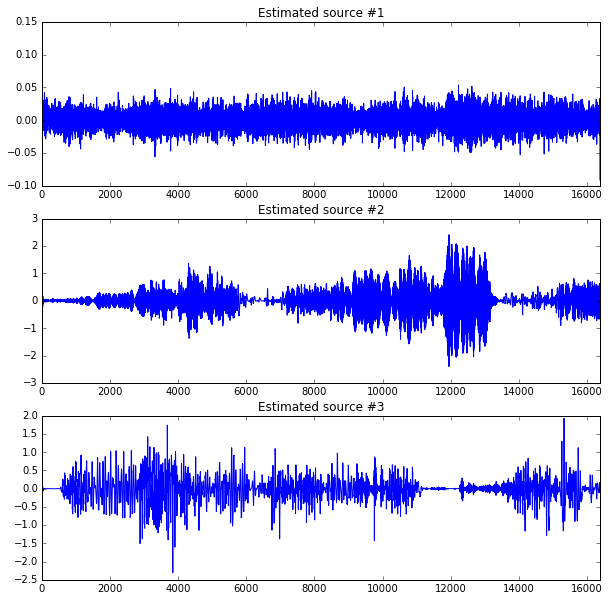One can listen to the recovered sources.

In :
i = 1
from IPython.display import Audio
Audio(x[:,i], rate = 15000)

Out: